##### The Japanese Bonsai specialist
Direct order Contact Help / Services Newsletter# Plastic watering can 3 litres

› Bonsai tools › Watering equipment › Copper watering can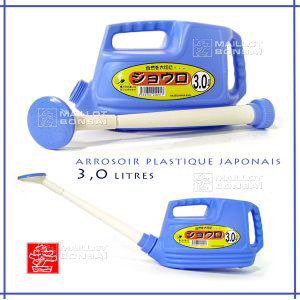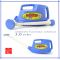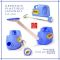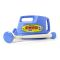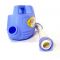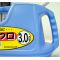ref. : 4344

13,00

This item is temporarily unavailableNotify availability

###### Description

Length of the spout without its rosette 250 mm. Weight empty 250 grams. Imported from Japan, this light-weight plastic watering can, with rosette, can hold 3 litres. It’s highly rated for dispensing liquid fertiliser solutions.

How to assemble it:

First make sure that the small white ring is on the base of the spout (BELOW NOT ABOVE the screw-on part), you’ll see a groove below the lip where it fits into. This seal will keep the spout watertight. Next you pass the blue ring over the top of the spout and screw it onto the can. (If the white ring is in the wrong place then the spout will leak water but when properly assembled the watering can works perfectly).

Interchangeable copper nozzle can also be added to this model. Reference 4350/4351

#watering 6.8 #spout 5.5 #plastic 3.7 #copper 3.6 #litres 3.6 #ring 3.4 #this 3.4 #rosette 2.7 #weight 2.6 #interchangeable 2.5

Formule
(( ROUND((CHAR_LENGTH(b.article_nom)-CHAR_LENGTH(REPLACE(b.article_nom, 'spout', '')))/LENGTH('spout')) + ROUND((CHAR_LENGTH(b.article_description)-CHAR_LENGTH(REPLACE(b.article_description, 'spout', '')))/LENGTH('spout')) ) * 5.5) + (( ROUND((CHAR_LENGTH(b.article_nom)-CHAR_LENGTH(REPLACE(b.article_nom, 'watering', '')))/LENGTH('watering')) + ROUND((CHAR_LENGTH(b.article_description)-CHAR_LENGTH(REPLACE(b.article_description, 'watering', '')))/LENGTH('watering')) ) * 4.8) + (( ROUND((CHAR_LENGTH(b.article_nom)-CHAR_LENGTH(REPLACE(b.article_nom, 'plastic', '')))/LENGTH('plastic')) + ROUND((CHAR_LENGTH(b.article_description)-CHAR_LENGTH(REPLACE(b.article_description, 'plastic', '')))/LENGTH('plastic')) ) * 3.7) + (( ROUND((CHAR_LENGTH(b.article_nom)-CHAR_LENGTH(REPLACE(b.article_nom, 'litres', '')))/LENGTH('litres')) + ROUND((CHAR_LENGTH(b.article_description)-CHAR_LENGTH(REPLACE(b.article_description, 'litres', '')))/LENGTH('litres')) ) * 3.6) + (( ROUND((CHAR_LENGTH(b.article_nom)-CHAR_LENGTH(REPLACE(b.article_nom, 'this', '')))/LENGTH('this')) + ROUND((CHAR_LENGTH(b.article_description)-CHAR_LENGTH(REPLACE(b.article_description, 'this', '')))/LENGTH('this')) ) * 3.4) + (( ROUND((CHAR_LENGTH(b.article_nom)-CHAR_LENGTH(REPLACE(b.article_nom, 'ring', '')))/LENGTH('ring')) + ROUND((CHAR_LENGTH(b.article_description)-CHAR_LENGTH(REPLACE(b.article_description, 'ring', '')))/LENGTH('ring')) ) * 3.4) + (( ROUND((CHAR_LENGTH(b.article_nom)-CHAR_LENGTH(REPLACE(b.article_nom, 'rosette', '')))/LENGTH('rosette')) + ROUND((CHAR_LENGTH(b.article_description)-CHAR_LENGTH(REPLACE(b.article_description, 'rosette', '')))/LENGTH('rosette')) ) * 2.7) + (( ROUND((CHAR_LENGTH(b.article_nom)-CHAR_LENGTH(REPLACE(b.article_nom, 'weight', '')))/LENGTH('weight')) + ROUND((CHAR_LENGTH(b.article_description)-CHAR_LENGTH(REPLACE(b.article_description, 'weight', '')))/LENGTH('weight')) ) * 2.6) + (( ROUND((CHAR_LENGTH(b.article_nom)-CHAR_LENGTH(REPLACE(b.article_nom, 'screw', '')))/LENGTH('screw')) + ROUND((CHAR_LENGTH(b.article_description)-CHAR_LENGTH(REPLACE(b.article_description, 'screw', '')))/LENGTH('screw')) ) * 2.5) + (( ROUND((CHAR_LENGTH(b.article_nom)-CHAR_LENGTH(REPLACE(b.article_nom, 'white', '')))/LENGTH('white')) + ROUND((CHAR_LENGTH(b.article_description)-CHAR_LENGTH(REPLACE(b.article_description, 'white', '')))/LENGTH('white')) ) * 2.5)

## Secure payment## Delivery

Our logistic partners :04 74 55 23 48
Pépinière MAILLOT-BONSAÏ
Le Bois Frazy
01990 RELEVANT - FRANCE
on appointment